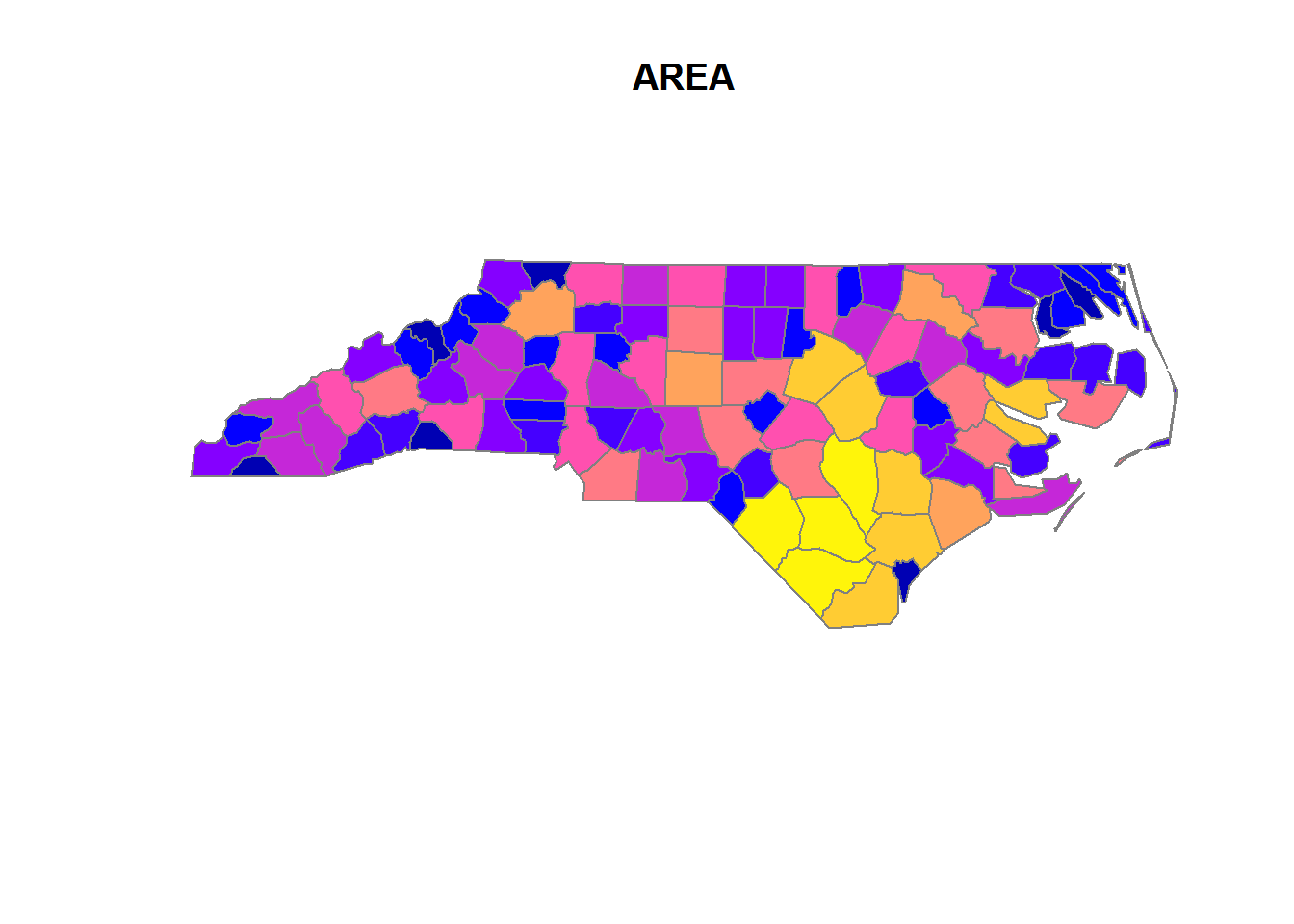# 20 Spatial data

## 20.1 IO

1. How to load/read and save/write spatial data?
``````library(sf)
## Linking to GEOS 3.5.1, GDAL 2.1.3, proj.4 4.9.2, lwgeom 2.3.2 r15302
nc <- st_read(system.file("shape/nc.shp", package="sf"), quiet = TRUE)
# st_write(nc, "nc.shp")
# see st_read_db() and st_write_db() for spatial databases
# look carefully through all of the arguments (especially layers,
# stringsAsFactors, geometry_column, type, quiet)
# in case of specific problems see:
# https://edzer.github.io/sfr/articles/sf2.html
#   ____________________________________________________________________________``````

## 20.2 Objects

1. What are the classes of sf-objects?
``````# the data.frame
class(nc)
#>  "sf"         "data.frame"

# the geometry list-column
class(st_geometry(nc))
#>  "sfc_MULTIPOLYGON" "sfc"

# the individual feature geometries
lapply(st_geometry(dplyr::slice(nc, 1:2)), class)
#> []
#>  "XY"           "MULTIPOLYGON" "sfg"
#>
#> []
#>  "XY"           "MULTIPOLYGON" "sfg"``````
1. Which methods are avaliable for sf-objects?
``````methods(class = "sf")
#>   \$<-               [                 [[<-
#>   aggregate         anti_join         arrange_
#>   cbind             distinct_         filter_
#>  full_join         group_by_         inner_join
#>  left_join         merge             mutate_
#>  plot              print             rbind
#>  rename_           right_join        sample_frac
#>  sample_n          select_           semi_join
#>  slice_            st_agr            st_agr<-
#>  st_as_sf          st_bbox           st_boundary
#>  st_buffer         st_cast           st_centroid
#>  st_convex_hull    st_coordinates    st_crs
#>  st_crs<-          st_difference     st_geometry
#>  st_geometry<-     st_intersection   st_is
#>  st_line_merge     st_make_valid     st_polygonize
#>  st_precision      st_segmentize     st_set_precision
#>  st_simplify       st_sym_difference st_transform
#>  st_triangulate    st_union          st_voronoi
#>  st_zm             summarise_        transmute_
#> see '?methods' for accessing help and source code

methods(class = 'sfc')
#>   [                 c                 format
#>   print             st_as_binary      st_as_text
#>   st_bbox           st_boundary       st_buffer
#>  st_cast           st_centroid       st_convex_hull
#>  st_coordinates    st_crs            st_crs<-
#>  st_difference     st_geometry       st_intersection
#>  st_is             st_line_merge     st_make_valid
#>  st_polygonize     st_precision      st_segmentize
#>  st_set_precision  st_simplify       st_sym_difference
#>  st_transform      st_triangulate    st_union
#>  st_voronoi        st_zm             str
#>  summary           type_sum
#> see '?methods' for accessing help and source code

methods(class = 'sfg')
#>   as.matrix         c                 format
#>   head              plot              print
#>   st_as_binary      st_as_text        st_boundary
#>  st_buffer         st_centroid       st_convex_hull
#>  st_coordinates    st_difference     st_geometry
#>  st_intersection   st_is             st_line_merge
#>  st_make_valid     st_polygonize     st_segmentize
#>  st_simplify       st_sym_difference st_transform
#>  st_triangulate    st_union          st_voronoi
#>  st_zm
#> see '?methods' for accessing help and source code``````
1. What sf-geometry types are available, how do you initialize them and what types are the internally?
``````# internal types:
# - point (atomic)
# - linestring (matrix)
# - everything else (list)

## common geometries
# point
st_point(c(1,2))
# linestring
st_linestring(rbind(c(0.2,3), c(0.2,4), c(1,4.8), c(2,4.8)))
# polygon
st_polygon(list(
rbind(c(0,0), c(1,0), c(3,2), c(2,4), c(1,4), c(0,0)),
rbind(c(1,1), c(1,2), c(2,2), c(1,1))))
# multipoint
st_multipoint(rbind(c(3.2,4), c(3,4.6), c(3.8,4.4), c(3.5,3.8), c(3.4,3.6)))
# multilinestring
st_multilinestring(list(rbind(c(0.2,3), c(0.2,4), c(1,4.8), c(2,4.8)),
rbind(c(0,4.4), c(0.6,5))))
# multipolygon
st_multipolygon(list(
list(rbind(c(0,0), c(1,0), c(3,2), c(2,4), c(1,4), c(0,0)),
rbind(c(1,1), c(1,2), c(2,2), c(1,1))),
list(rbind(c(3,0), c(4,0), c(4,1), c(3,1), c(3,0)),
rbind(c(3.3,0.3), c(3.8,0.3), c(3.8,0.8), c(3.3,0.8), c(3.3,0.3))[5:1,]),
list(rbind(c(3,3), c(4,2), c(4,3), c(3,3)))))

# geometrycollection
st_sfc(st_geometrycollection(list(st_point(1:2))),
st_geometrycollection(list(st_linestring(matrix(1:4,2)))))
# geometry
st_sfc(st_point(1:2), st_linestring(matrix(1:4,2))) # like the latter, but as a "superclass"

## rare geometries
# circularstring
# compoundcurve
# curvepolygon
# multicurve
# multisurface
# curve
# surface
# polyhedral surface
# tin
# triangle``````
1. What are the dimensions of those objects?
``````x = st_sfc(
st_point(0:1),
st_linestring(rbind(c(0,0),c(1,1))),
st_polygon(list(rbind(c(0,0),c(1,0),c(0,1),c(0,0)))),
st_multipoint(),
st_linestring(),
st_geometrycollection())
st_dimension(x, NA_if_empty = FALSE)
#>  0 1 2 0 1 0``````
1. How to initialize these objects from well known text?
``````st_as_sfc("POINT(0 1)")
#> Geometry set for 1 feature
#> geometry type:  POINT
#> dimension:      XY
#> bbox:           xmin: 0 ymin: 1 xmax: 0 ymax: 1
#> epsg (SRID):    NA
#> proj4string:    NA
#> POINT(0 1)``````
1. How to initialize an sf-object and bring it into coordinate reference systems (crs)?
``````# attributes of sfc obejects to store a crs: epsg, proj4string
# theses must be the same for all elements of an sfc-object, but can also be NA.
# -proj4string is a generic, string-based description of crs
# - epsg integer values refer to particular well known crs, that might also
# improve over time

st_sf(points = st_sfc(st_point(c(0, 0)),
st_point(c(0, 1)),
crs = 4326))
#> Simple feature collection with 2 features and 0 fields
#> geometry type:  POINT
#> dimension:      XY
#> bbox:           xmin: 0 ymin: 0 xmax: 0 ymax: 1
#> epsg (SRID):    4326
#> proj4string:    +proj=longlat +datum=WGS84 +no_defs
#>       points
#> 1 POINT(0 0)
#> 2 POINT(0 1)
# also use:
# use st_crs() and st_transform()``````
1. How to coerce data.frames to sf?
``# use st_as_sf``
1. How to create and identify empty geometries?
``````st_geometrycollection()
#> GEOMETRYCOLLECTION()
length(st_geometrycollection())
#>  0``````
1. How to identify unconnected parts of geometries?
``````which(sapply(st_geometry(nc), length) > 1)
#>   4 56 57 87 91 95``````
1. How to switch between well-known text and well-known binary encoding?
``````x <- st_linestring(matrix(10:1,5))
st_as_text(x)
#>  "LINESTRING(10 5, 9 4, 8 3, 7 2, 6 1)"
st_as_binary(x)
#>   01 02 00 00 00 05 00 00 00 00 00 00 00 00 00 24 40 00 00 00 00 00 00
#>  14 40 00 00 00 00 00 00 22 40 00 00 00 00 00 00 10 40 00 00 00 00 00
#>  00 20 40 00 00 00 00 00 00 08 40 00 00 00 00 00 00 1c 40 00 00 00 00
#>  00 00 00 40 00 00 00 00 00 00 18 40 00 00 00 00 00 00 f0 3f``````
1. How to control geometries for simplicity and validity?
``````st_is_valid(st_as_sfc("POLYGON((0 0, 0 10, 10 0, 10 10, 0 0))"))
#> Warning in eval(substitute(expr), envir, enclos): Self-intersection at or
#> near point 5 5
#>  FALSE
st_is_simple(st_as_sfc("POLYGON((0 0, 0 10, 10 0, 10 10, 0 0))"))
#>  TRUE
plot(st_as_sfc("POLYGON((0 0, 0 10, 10 0, 10 10, 0 0))"))``````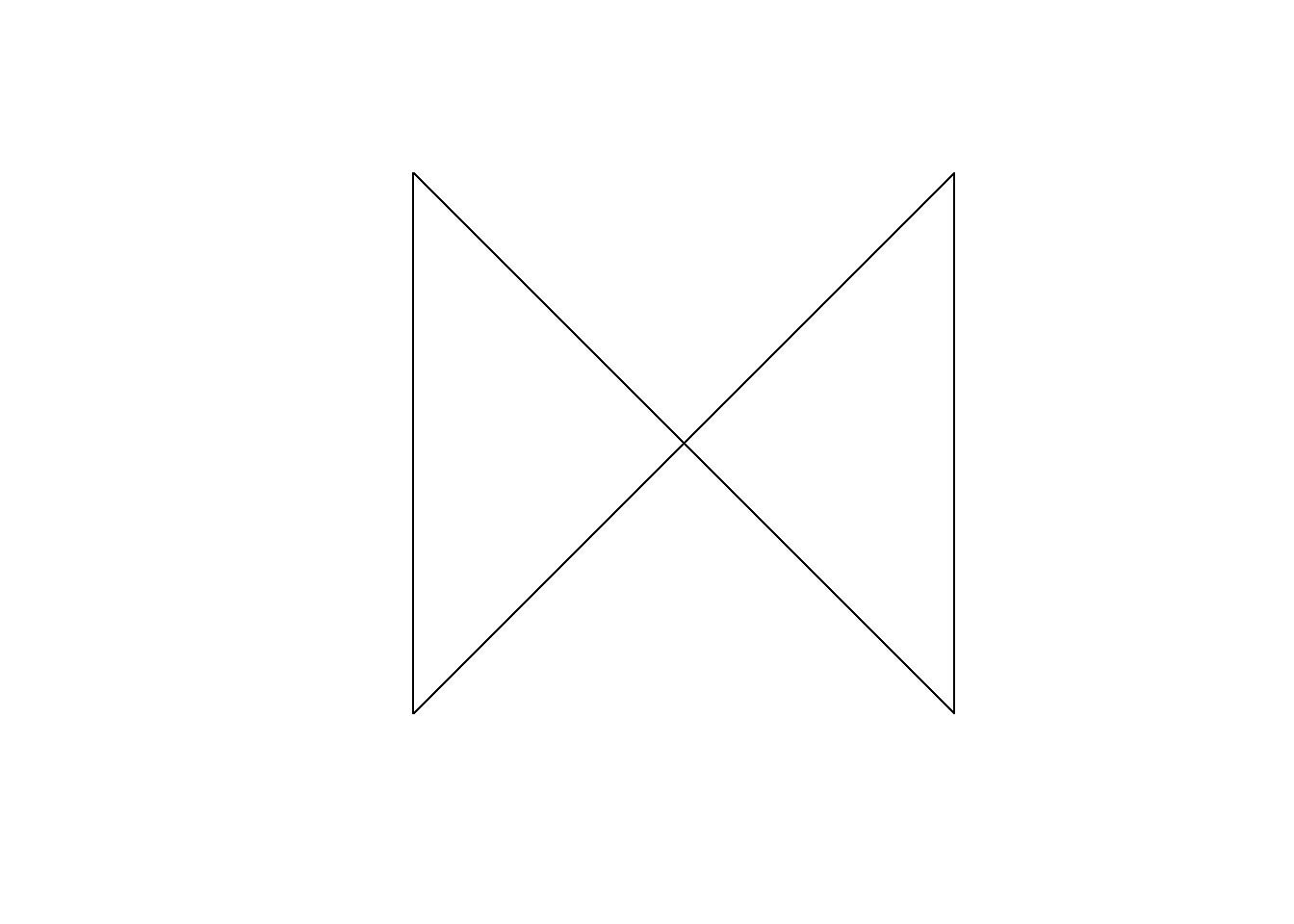1. How to create a grid manually?
``````library(dplyr)
lat <- seq(from = 1, to = 50, length.out = 100)
long <- seq(from = 1, to = 50, length.out = 100)
grid <- tidyr::expand(tibble(lat = lat, long = long), lat, long)
grid <- grid %>%
mutate(points = purrr::map2(lat, long,
~st_geometrycollection(list(st_point(c(.x,.y))))))
grid <- grid %>% mutate(points = st_sfc(points))
grid <- st_sf(grid, stringsAsFactors = FALSE,
sf_column_name = "points")
plot(grid %>% select(points))``````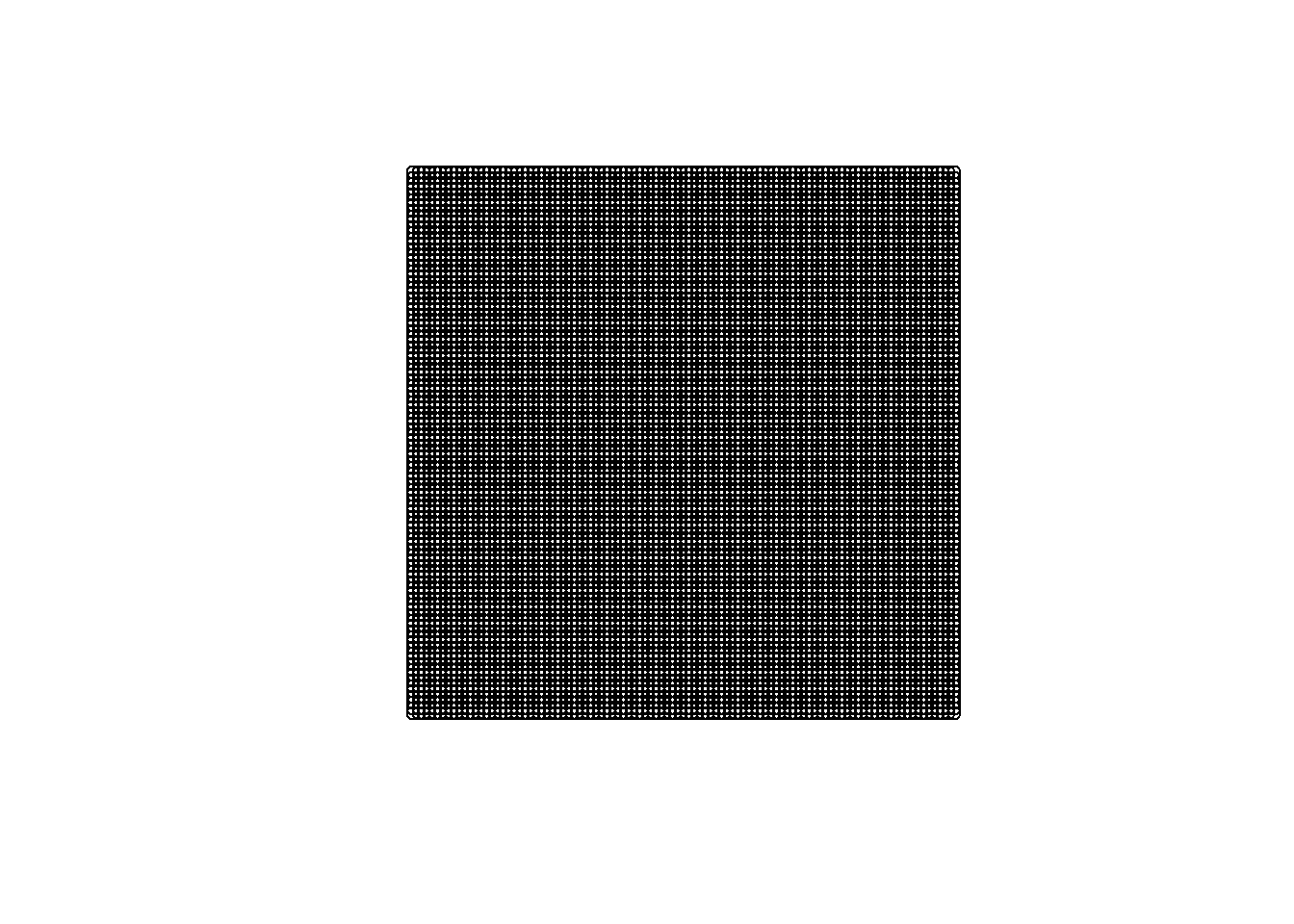How to set aggregations?

``````# while initializing
nc <- st_read(system.file("shape/nc.shp", package="sf"),
agr = c(AREA = "aggregate", PERIMETER = "aggregate", CNTY_ = "identity",
CNTY_ID = "identity", NAME = "identity", FIPS = "identity", FIPSNO = "identity",
CRESS_ID = "identity", BIR74 = "aggregate", SID74 = "aggregate", NWBIR74 = "aggregate",
BIR79 = "aggregate", SID79 = "aggregate", NWBIR79 = "aggregate"))
#> Reading layer `nc' from data source `D:\R\Projects\Tidyverse-Cookbook\packrat\lib\x86_64-w64-mingw32\3.3.3\sf\shape\nc.shp' using driver `ESRI Shapefile'
#> converted into: POLYGON
#> Simple feature collection with 100 features and 14 fields
#> Attribute-geometry relationship: 0 constant, 8 aggregate, 6 identity
#> geometry type:  MULTIPOLYGON
#> dimension:      XY
#> bbox:           xmin: -84.32385 ymin: 33.88199 xmax: -75.45698 ymax: 36.58965
#> epsg (SRID):    4267
#> proj4string:    +proj=longlat +datum=NAD27 +no_defs``````

## 20.3 Arithmetics

1. How to calculate with simple features?
``````st_polygon(list(rbind(c(0,0),c(1,0),c(1,1),c(0,1),c(0,0)))) - 0.5
#> POLYGON((-0.5 -0.5, 0.5 -0.5, 0.5 0.5, -0.5 0.5, -0.5 -0.5))
st_point(c(0,0)) + 1
#> POINT(1 1)``````
1. How to calculate distances?
``````x = st_transform(nc, 32119)
st_distance(x[c(1,4,22),], x[c(1, 33,55,56),], dist_fun = geosphere::distGeo)
#> Units: m
#>           [,1]     [,2]      [,3]     [,4]
#> [1,]      0.00 312184.9 128341.85 475623.3
#> [2,] 440561.15 114939.7 590434.80      0.0
#> [3,]  18944.03 352719.1  78756.89 517527.8
line = st_sfc(st_linestring(rbind(c(30,30), c(40,40))), crs = 4326)
st_length(line)
#> 1434649 m``````

## 20.4 Plots

1. How does the plot method and its `add` argument work?
``plot(nc[1,1], col = 'grey')``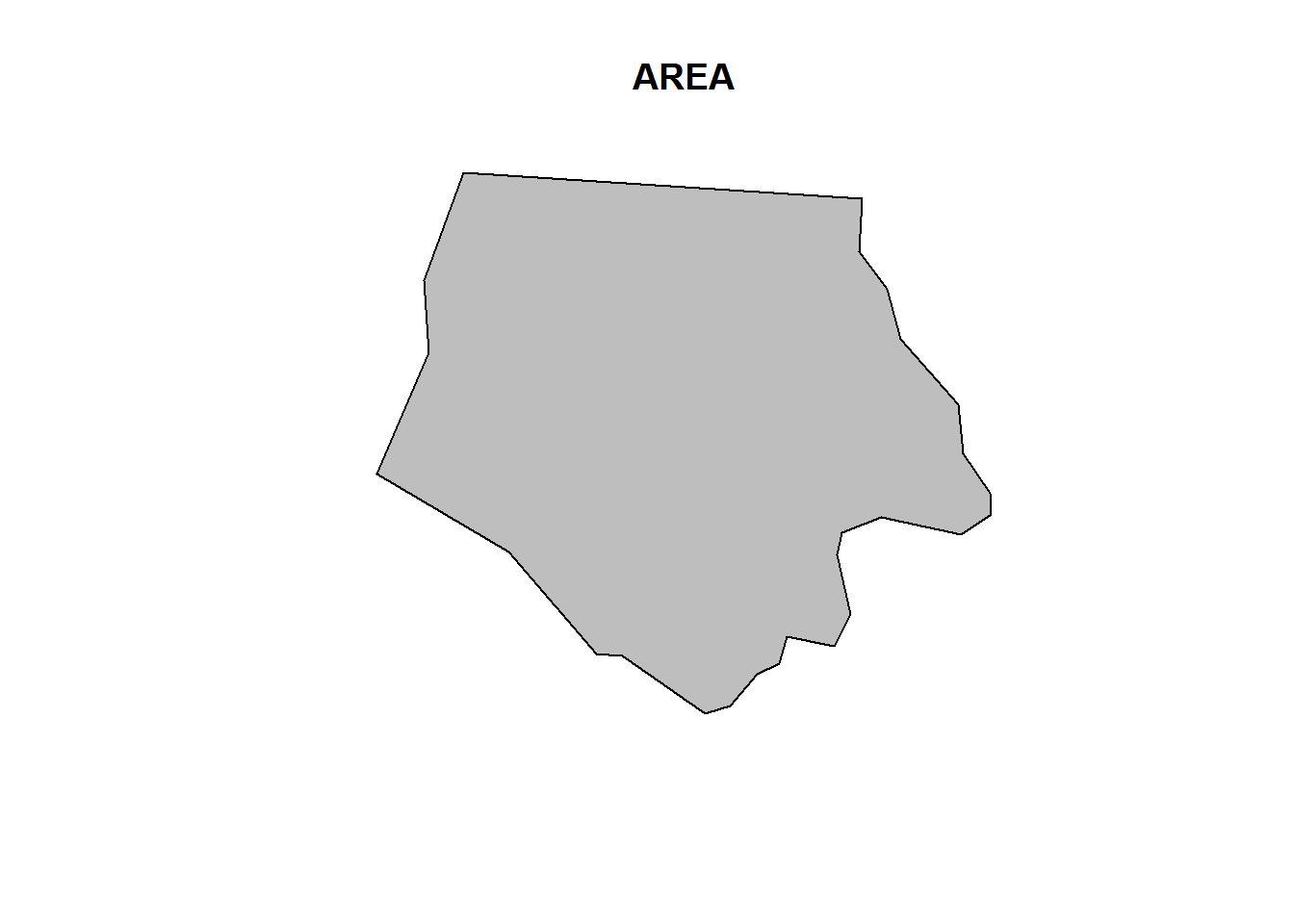``````plot(nc[1,1], col = 'grey')
plot(nc, add = TRUE)``````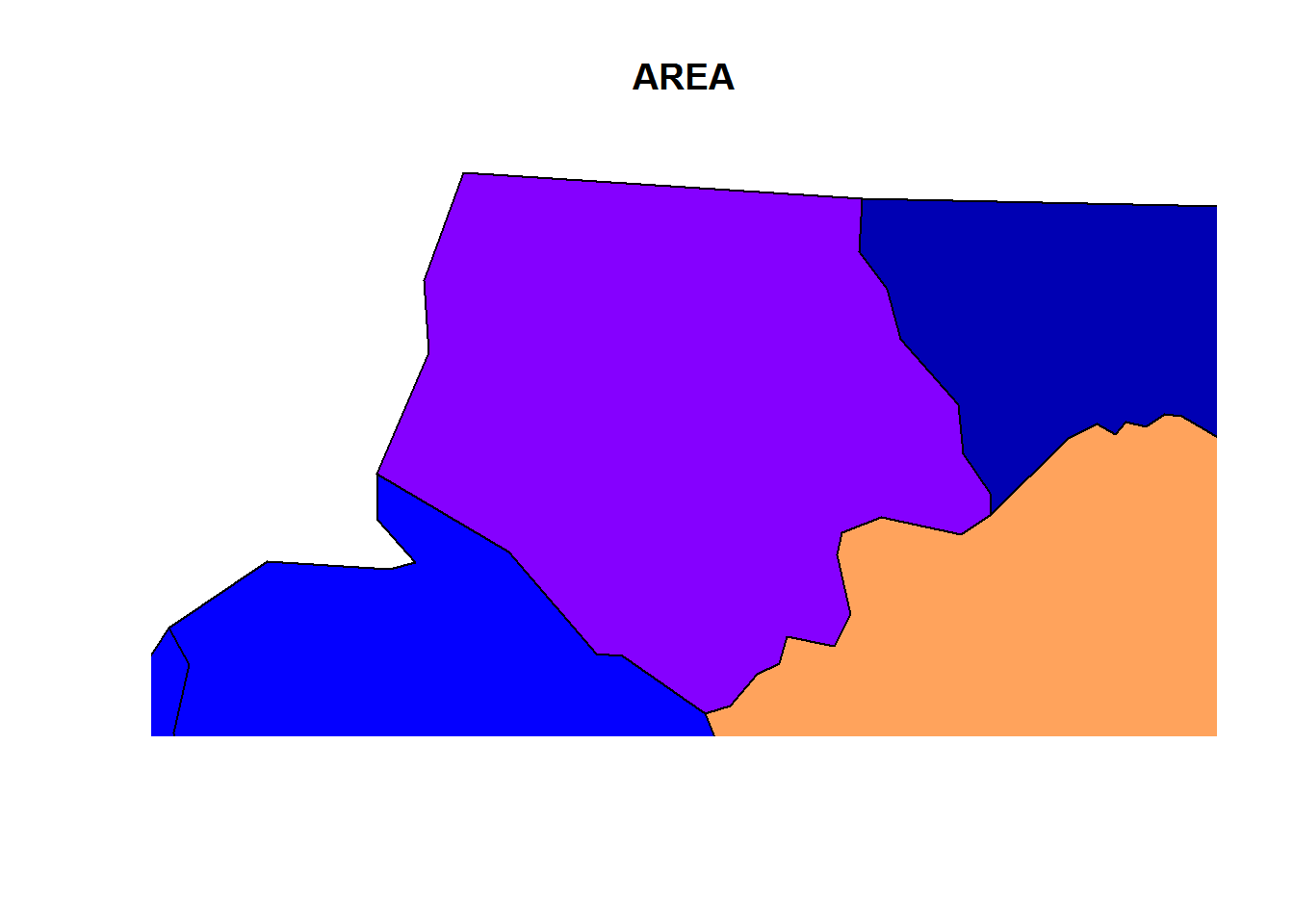``````
plot(nc)
plot(nc[1,1], col = 'grey', add = TRUE)``````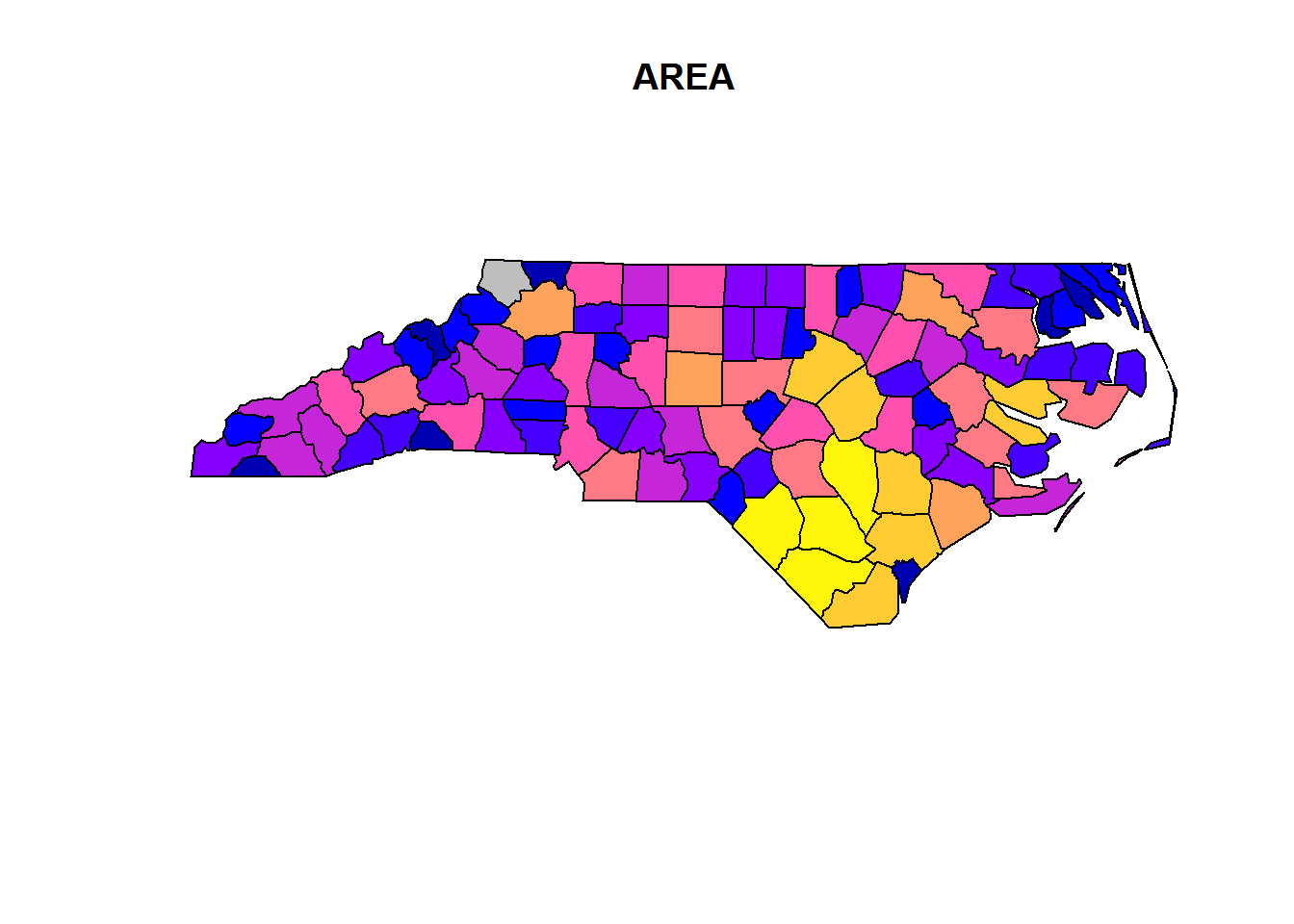1. Some differenct plot options
``plot(nc)``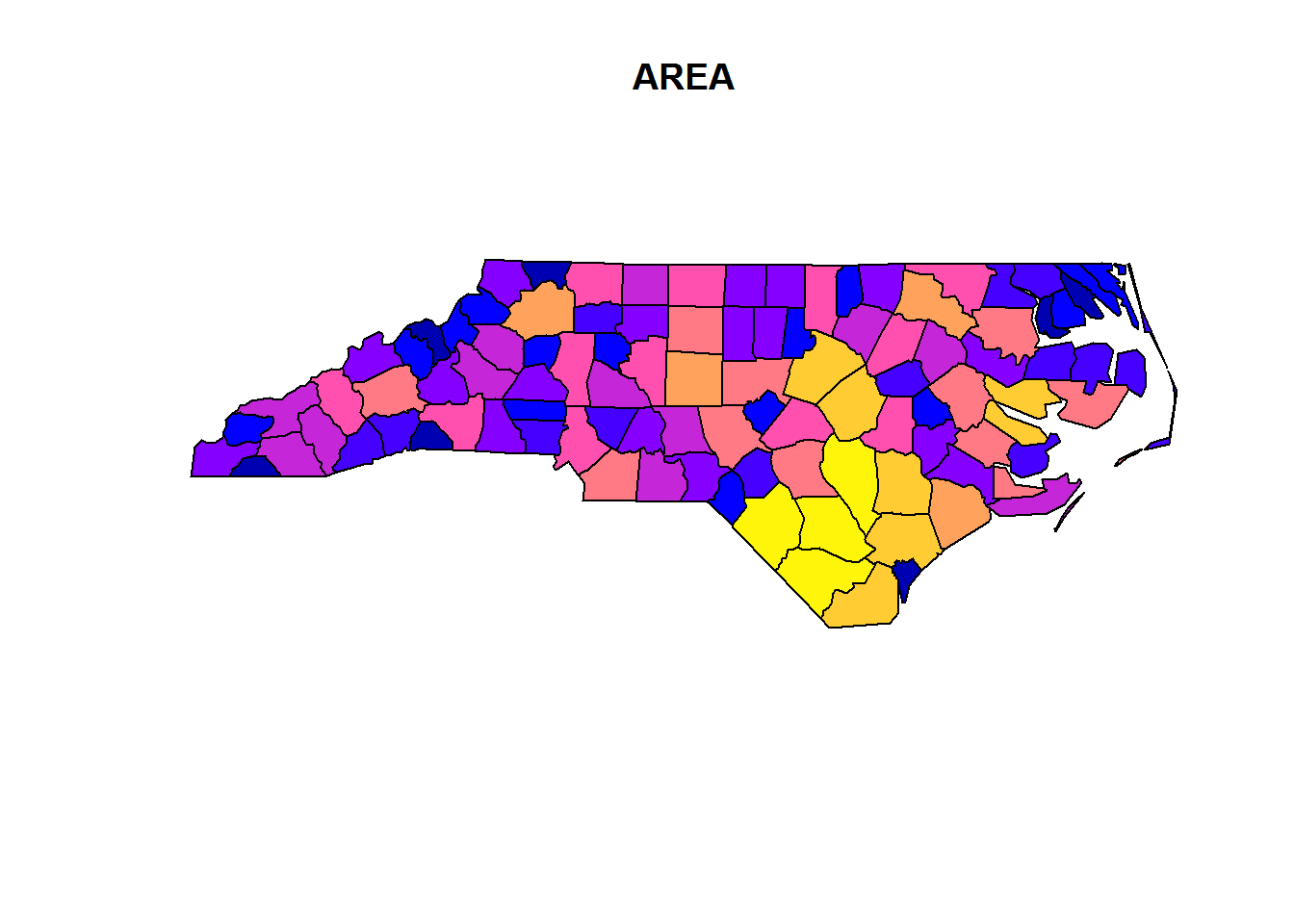``plot(st_convex_hull(nc))``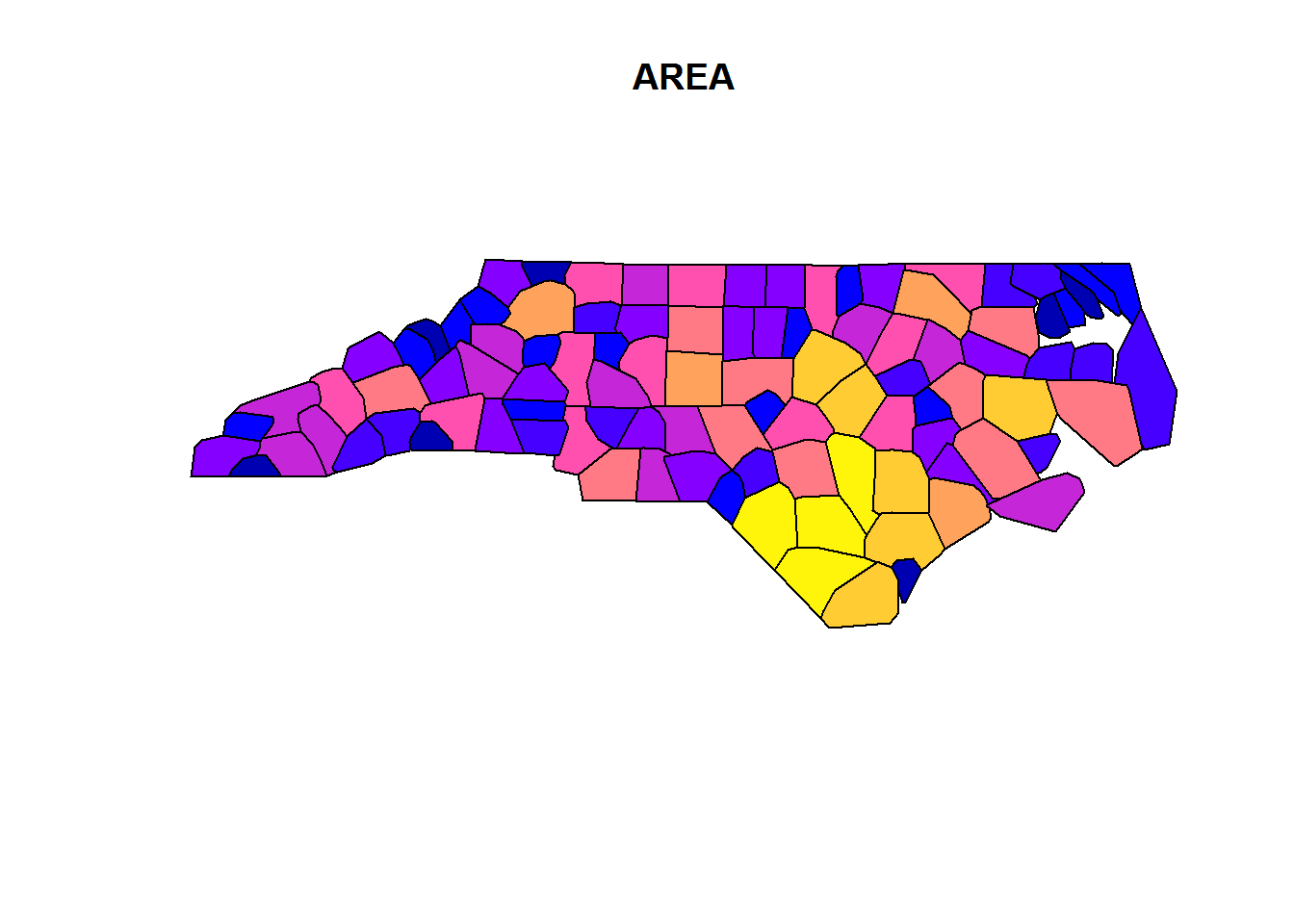``plot(nc, border = grey(.5))``